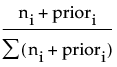Probi =where the summation is across all response levels; ni is the number of observations at the node for the ith response level; and priori is the prior probability for the ith response level, calculated as:
priori = λpi+ (1-λ)Pi
where pi is the priori from the parent node, Pi is the Probi from the parent node, and λ is a weighting factor currently set at 0.9.

Help created on 9/19/2017